Identifying fractions
Identifying fractions on a number line
Compare and Order Fractions
With Common Denominators
Subtracting Fractions With Common Denominators
Subtracting Fractions With Uncommon Denominators
1001/2

100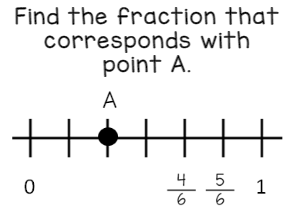2/6

100

Compare the fractions using <, >, or =

5/6     4/6

5/6  >  4/6

100

Add the fractions:   2/5  +  3/5

5/5 which is 1 whole

100

Subtract the fractions:   4/7 - 1/7

3/7

100

3/4 + 5/7

41/48

100

Subtract

4/5 - 1/3

7/15

200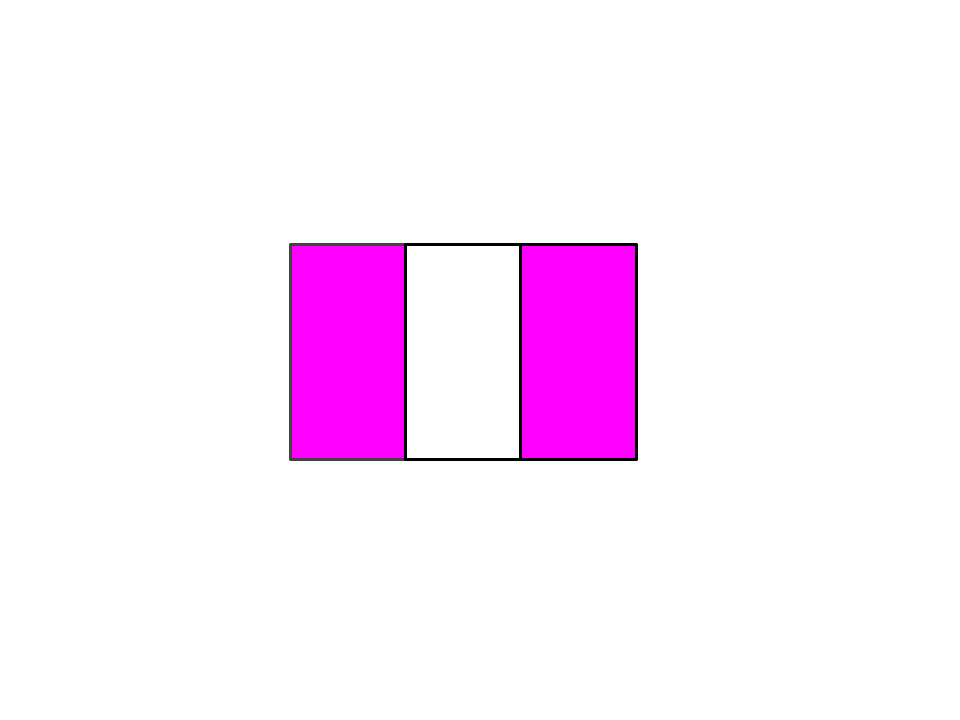2/3

200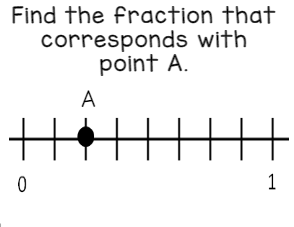2/8   same as 1/4

200

Compare the fractions using <, >, or =.

3/4     3/8

3/4  >  3/5

200

11/20

200

Subtract the fractions:  19/20  -  6/20

13/20

200

3/8 + 4/5

47/40   which is 1 and 7/40

200

Subtract

8/11- 3/5

7/55

300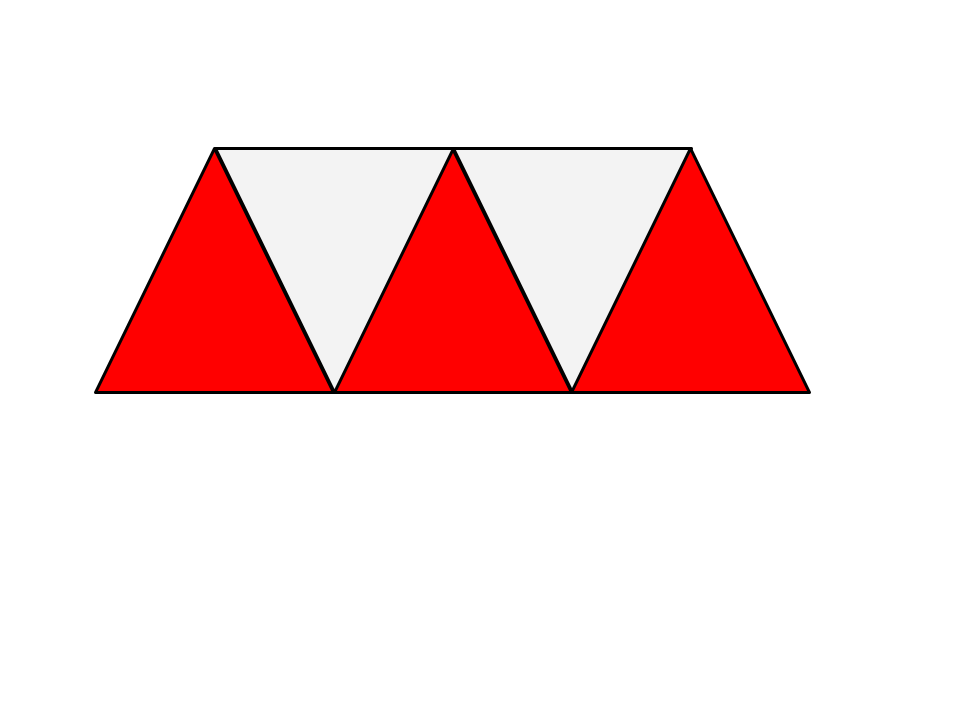3/5

300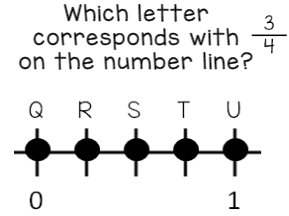T

300

Compare the fractions using <, >, or =.

10/20   10/12

10/20 < 10/12

300

9/8 or 1 1/8

300

Subtract the fractions:   8/14 -  6/14

2/14   which simplifies to 1/7

300

1/3 + 1/4

7/12

300

Subtract

3/4 - 1/2

2/8 which simplifies to 1/4

400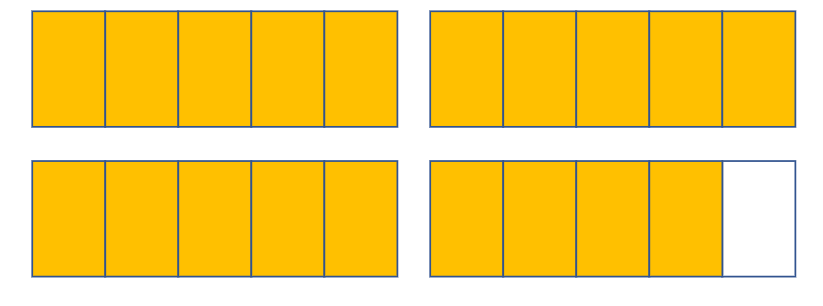3 4/5

400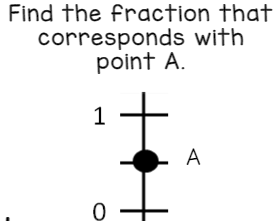1/2

400

Compare the fractions using <, >, or =.

1/2   5/10

1/2 = 5/10
400

5/10 or 1/2

400

6/9- 3/9

3/9  which simplifies to 1/3

400

1/3 +  4/5

17/15   which is also 1 and 2/15

400

Subtract

2/3 - 1/4

5/12

500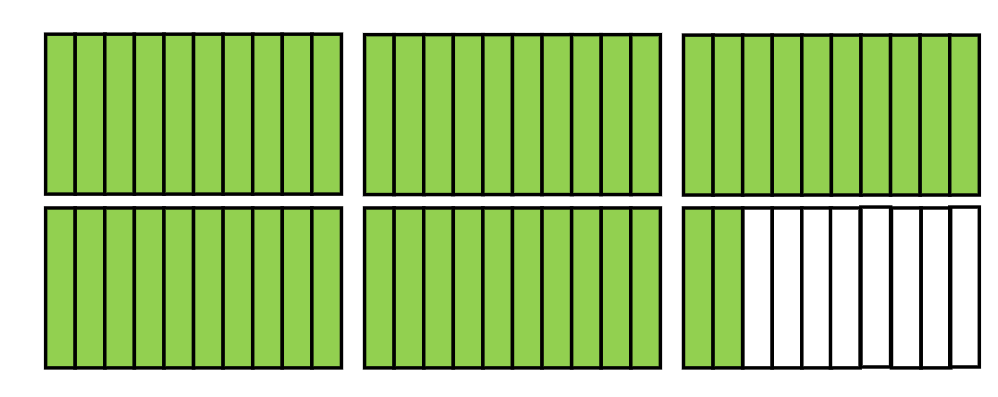5 2/10 same as 5 1/5

500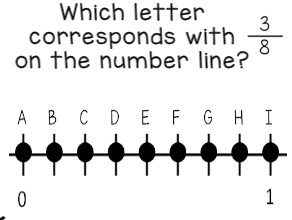D

500

Compare the fractions using >, <, =

2/10     20/100

2/10 = 20/100

500

1/5 + 2/5 =

3/5

500

2/4- 1/4 =

1/4

500

3/5 +   7/8

59/40  which can be written as 1 and 19/40

500

Subtract

1/2- 1/9

7/18

Click to zoom